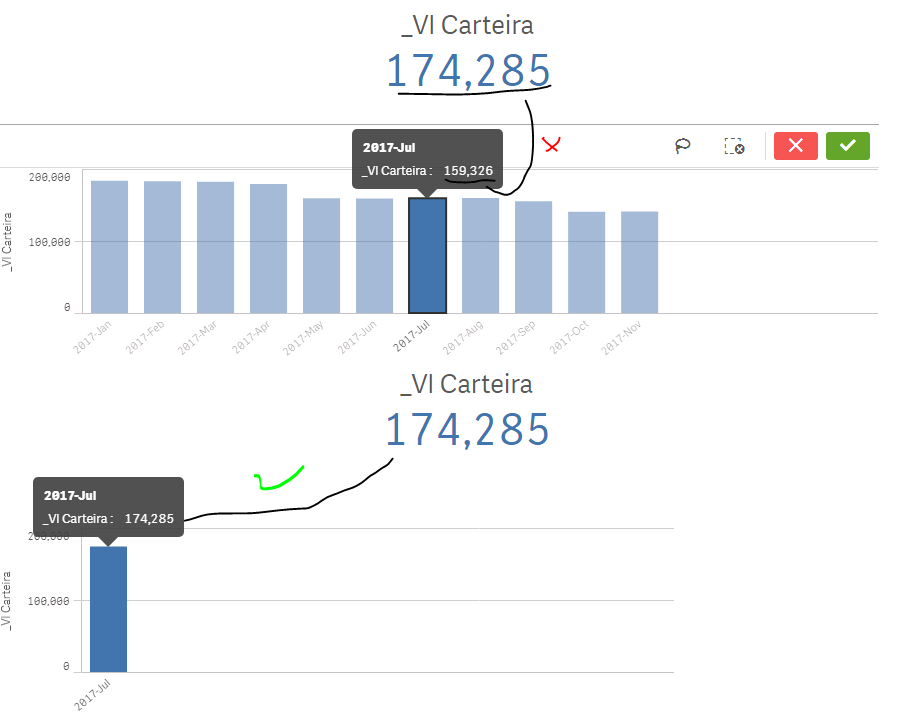# New to Qlik Sense

If you’re new to Qlik Sense, start with this Discussion Board and get up-to-speed quickly.

Announcements
Skip the ticket, Chat with Qlik Support instead for instant assistance.
cancel
Showing results for
Did you mean:Creator II

## Measure evaluating by the max dimension

This is hard to explain, but I need to force the values on a chart to calculate based on their domain.

Here is the Set Analysis for "_Vl Carteira", which basically excludes the sum when either DtPrej or DtPrejGer are smaller than DtRef

Sum({(\$-<DtPrej = {"\$(= '<' & DtRef)"}> - <DtPrejGer = {"\$(= '<' & DtRef)"}>)

*

<ProdutoFinanceiro -={'XYZ', 'ASD'},

DtRef = {"=Day(DtRef)=1"}>}

Saldo)

As you can see by the behavior below, the measure seems to calculate based on the max DtRef available (in this case 2017-Nov) However, it calculates correctly when only its own DtRef.YearMonth dimension is selected (matches the KPI object value).

Can anyone help me with the expression? Maybe something with Aggr()?

(And yes, I do realize that putting a flag on my script for the date comparison would be a better solution.)1 Solution

Accepted SolutionsMVP

Try this

Sum(Aggr(If(Alt(DtPrej, MakeDate(9999, 12, 31)) > DtRef and Alt(DtPrejGer, MakeDate(9999, 12, 31)) > DtRef and Day(DtRef) = 1, Sum(Saldo)), DtPrej, DtPrejGer, DtRef))

9 RepliesMVP

Difficult to say what the exact expression needs to be, but I feel that you might need to use Aggr() function rather than set analysis because set analysis is evaluated once per chart and from what you are showing, it almost seems like you don't want that. You want dimension wise evaluation of set analysis, which is only possible using Aggr() functionCreator II
Author

Here's what I've tried, with the same results. Any ideas?

Sum(Aggr(

Sum({(\$-<DtPrej = {"\$(= '<' & DtRef)"}> - <DtPrejGer = {"\$(= '<' & DtRef)"}>)

*

<ProdutoFinanceiro -={'XYZ', 'ASD'},

DtRef = {"=Day(DtRef)=1"}>}

Saldo), DtRef))MVP

You still have set analysis in there, I doubt that will work alsoCreator II
Author

Can I not combine Aggr and Set Analysis?

I've been trying to follow these guidelines: Pitfalls of the Aggr function

How can I replicate my set analysis conditions without using set analysis?MVP

You can, but for what you need, you need to convert your set analysis into Aggr()Creator II
Author

Any hints to get me started?MVP

Not sure what else to mention... more than I already did... May be if you share a sample, I might be able to help betterCreator II
Author

Hi Sunny,

See a sample app attached, with data and the measures.

In the graph, the values for 2017-Jul and 2017-Aug are ~40k lower than expected, since it is not considering PropID = 427242.

The KPI object displays the value correctly

Please let me know if you have any questions.MVP

Try this

Sum(Aggr(If(Alt(DtPrej, MakeDate(9999, 12, 31)) > DtRef and Alt(DtPrejGer, MakeDate(9999, 12, 31)) > DtRef and Day(DtRef) = 1, Sum(Saldo)), DtPrej, DtPrejGer, DtRef))Tags
Community Browser